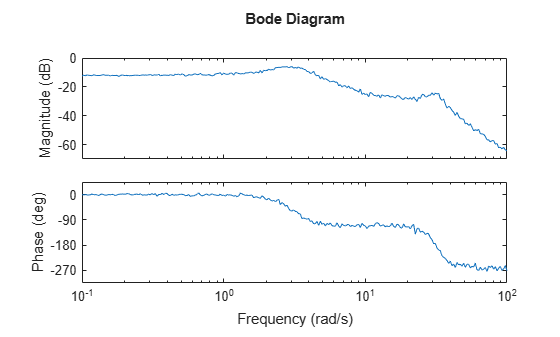## Frequency Response Data (FRD) Models

### Frequency Response Data

In the Control System Toolbox™ software, you can use `frd` models to store, manipulate, and analyze frequency response data. An `frd` model stores a vector of frequency points with the corresponding complex frequency response data you obtain either through simulations or experimentally.

For example, suppose you measure frequency response data for the SISO system you want to model. You can measure such data by driving the system with a sine wave at a set of frequencies ω1ω2, ,...,ωn, as shown:At steady state, the measured response yi(t) to the driving signal at each frequency ωi takes the following form:

`${y}_{i}\left(t\right)=a\mathrm{sin}\left({\omega }_{i}t+b\right),\text{ }i=1,\text{\hspace{0.17em}}\dots ,\text{\hspace{0.17em}}n.$`

The measurement yields the complex frequency response G at each input frequency:

`$G\left(j{\omega }_{i}\right)=a{e}^{jb},\text{ }i=1,\text{\hspace{0.17em}}\dots ,\text{\hspace{0.17em}}n.$`

You can do most frequency-domain analysis tasks on `frd` models, but you cannot perform time-domain simulations with them. For information on frequency response analysis of linear systems, see Chapter 8 of .

### Commands for Creating FRD Models

Use the following commands to create FRD models.

Command

Description

`frd`Create `frd` objects from frequency response data.
`frestimate` (Simulink Control Design)Create `frd` objects by estimating the frequency response of a Simulink® model. This approach requires Simulink Control Design™ software. See Offline Frequency Response Estimation (Simulink Control Design) for more information.

### Create Frequency Response Model from Data

This example shows how to create a single-input, single-output (SISO) frequency-response model using `frd`.

A frequency-response model stores a vector of frequency points with corresponding complex frequency response data you obtain either through simulations or experimentally. Therefore, if you measure the frequency response of your system at a set of test frequencies, you can use the data to create a frequency response model.

Load the frequency response data in `AnalyzerData.mat`.

`load AnalyzerData`

This command loads the data into the MATLAB® workspace as the column vectors `freq` and `resp`. The variables `freq` and `resp` contain 256 test frequencies and the corresponding complex-valued frequency response points, respectively.

Create a frequency response model.

`sys = frd(resp,freq);`

`sys` is an `frd` model object that represents frequency response data.

You can use `frd` models with many frequency-domain analysis commands. For example, visualize the frequency response data using `bode`.

`bode(sys)`By default, the `frd` function assumes that the frequencies are in radians/second. To specify different frequency units, use the `TimeUnit` and `FrequencyUnit` properties of the `frd` model object. For example, set the frequency units to radians/minute.

```sys = frd(resp,freq,... "TimeUnit","min","FrequencyUnit","rad/TimeUnit");```